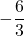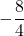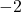## line F has a slope of. -6/3 and line G has a slope of -8/4. what can be determined about distinct lines F and G?

Question

line F has a slope of. -6/3 and line G has a slope of -8/4.
what can be determined about distinct lines F and G?

in progress 0
2 months 2021-07-28T13:36:15+00:00 1 Answers 1 views 0

1. Given:

Slope of line F =Slope of line G =To find:

The conclusion about distinct lines F and G.

Solution:

We have,

Slope of line F ==Slope of line G ==The slopes of lines F and G are equal and we know that the slopes of two parallel lines are always equal.

Therefore, the line F and line G are parallel to each other.